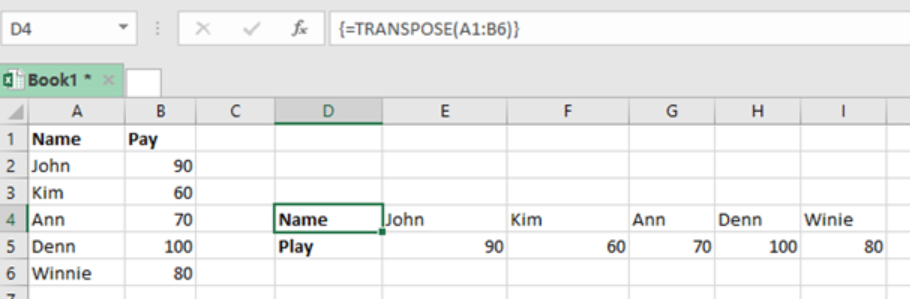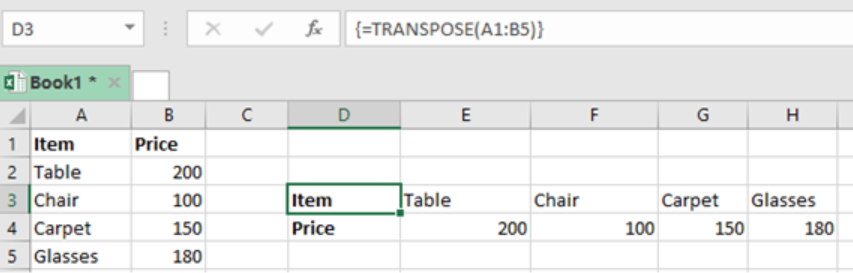Get instant live expert help with Excel or Google Sheets“My Excelchat expert helped me in less than 20 minutes, saving me what would have been 5 hours of work!”

#### Post your problem and you’ll get Expert help in seconds.

Your message must be at least 40 characters
Our professional Expert are available now. Your privacy is guaranteed.

# Excel TRANSPOSE Function

We can use the Excel TRANSPOSE function to ‘flip’ the orientation of a given range or array. This function changes the horizontal range to vertical or vertical to horizontal range. To understand how the Excel TRANSPOSE function works, read this post to the end.Figure 1: How to use Transpose function

## General syntax of the formula

`=TRANSPOSE (array)`

Where;

• Array- shows the array or range of value that you want to transpose

## How the formula works

• The function helps us to convert the vertical range of cells to horizontal range if cells
• It also converts horizontal range of cells to vertical range of cells

## How to use it

For the formula to work perfectly well, you need to enter it as an array formula, i.e. by pressing Control + Shift + Enter.

You need to specify the number of rows for the flipped range to be same as the column number in the original range.

Note that the first row becomes the first column, second row becomes second column and so on and so forth.

## ExampleFigure 2: Using TRANSPOSE function to flip range of values

Step 2: Select the cells where you want the transposed data to appear. Note that the number of rows at the point where you want to put the Transposed values should be equal to the number of columns in the original.

Step 3: Enter the formula in the formula bar

Step 4: Press Enter in an array form i.e. Ctrl + Shift + Enter

## Instant Connection to an Expert through our Excelchat Service

Most of the time, the problem you will need to solve will be more complex than a simple application of a formula or function. If you want to save hours of research and frustration, try our live Excelchat service! Our Excel Experts are available 24/7 to answer any Excel question you may have. We guarantee a connection within 30 seconds and a customized solution within 20 minutes.

### Did this post not answer your question? Get a solution from connecting with the expert.Another blog reader asked this question today on Excelchat:## Subscribe to Excelchat.coAnother blog reader asked this question today on Excelchat: